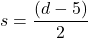## Diego’s age d is 5 more than 2 times his sister’s age s. This situation is represented by the equation d=2s+5. If we know Diego’s age,

Question

Diego’s age d is 5 more than 2 times his sister’s age s. This situation is represented by the equation d=2s+5. If we know Diego’s age, which equation will help us find his sister’s age?

in progress 0
4 weeks 2021-08-30T21:31:31+00:00 1 Answers 0 views 0

1. Answer:.
To find his sister’s age we can write the above equation as[we first subtract 5 from both sides and then divide both sides by 2]
Hence, the required equation:.